Open in App
Not now

# Find the coordinates of the points of trisection of the line segment joining the points A(2, – 2) and B(– 7, 4)

• Last Updated : 02 Dec, 2021

Mathematics is a subject that is associated with numbers and calculations. And, according to the type of calculation mathematics is divided into different branches like algebra, geometry, arithmetic, etc.

Geometry It is the branch of mathematics that deals with shape and their properties. Geometry that deals with points, lines, and planes in which coordinates are involved is called coordinate geometry.

### Coordinates

The location of any point on a plane can be expressed as (x, y) and these pairs are known as the coordinates, x is the horizontal value of a point on the plane. This value can also be called the x-coordinate or Abscissa, y is the vertical value of point on the plane. This value can be called the y-coordinate or ordinate. In coordinate geometry, the point is represented on the cartesian plane.

Cartesian plane

It is a plane formed by two perpendicular lines that is the x-axis (horizontal axis) and the y-axis (vertical axis). The position of a point in the Cartesian plane can be represented using the ordered pair (x, y).

### Section formula

If line A and B have coordinates  (x1, y1) and (x2, y2 ) respectively and C be a point that divides the line in the ratio m:n, then the coordinates of the point P is given as,

• When the ratio m:n is internal: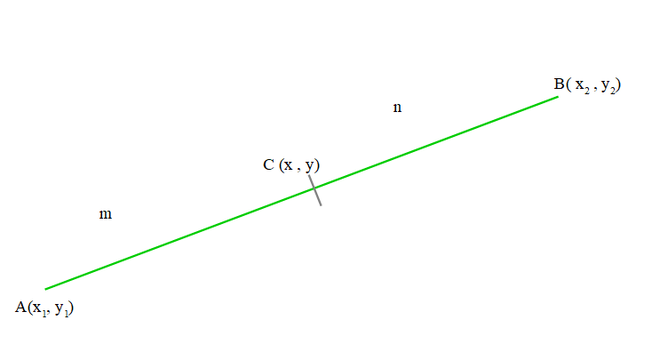1. x = (mx2 + nx1) / (m + n)
2. y = (my2 + ny1) / (m + n)
• When the ratio is external: When point lies outside the line segment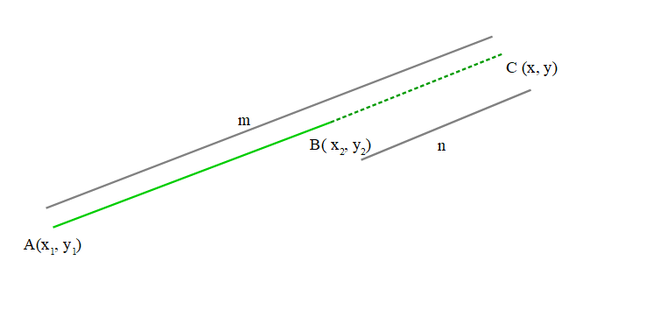1. x = (mx2  – nx1) / (m + n)
2. y = (my2 –  ny1) / (m + n)

### Find the coordinates of the points of trisection of the line segment joining the points A(2, – 2) and B(– 7, 4).

Solution:

Point of trisection means the points that can divide a line into three equal parts. According to the question we have a line segment joining A(2, -2) and B (-7, 4) and we have to find the points that can divide the line AB into three parts having an equal length. A line AB as shown in the diagram is given below,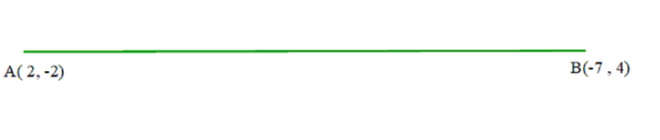Suppose C and D to be points of intersection,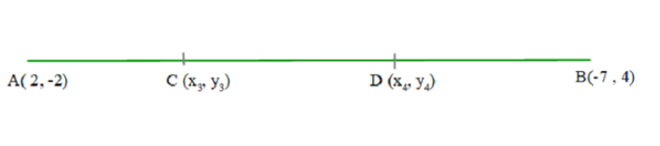So, according to the question, the coordinates of C and D are required.

In coordinate, section formula is used to find a point that divides a line into m:n ratio. According to the question, let C and D be the point of trisection having coordinates (x3, y3) and (x4, y4) respectively. Therefore,

AC = CD = DB

Then, point C will divide the line AB in ratio 1:2. Apply section formula to get the coordinate of point C.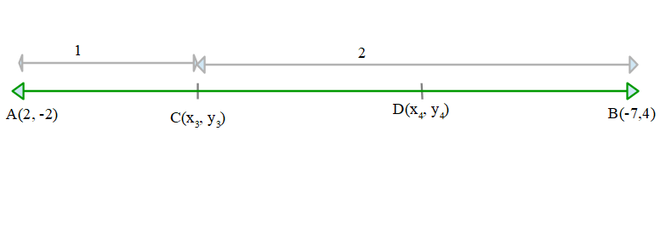Here,

m = 1, n= 2

x1 = 2, y1 = -2

x2 = -7, y2 = 4

Apply section formula:

x3 = (mx2 + nx1) /(m + n)

= ((1)(-7) + (2)(2)) / (1 + 2)

=(-7 + 4) / 3

= -3/3

= -1

y4 = (my2 + ny2) / (m + n)

= ((1)(4) + (2)(-2))/ (1+2)

= (4 – 4) / 3

= 0

Coordinate of C(x3, y4) = (-1, 0)

C and D divide AB into three equal parts, then CD = DB. Now using section formula or midpoint formula, calculate coordinate of D.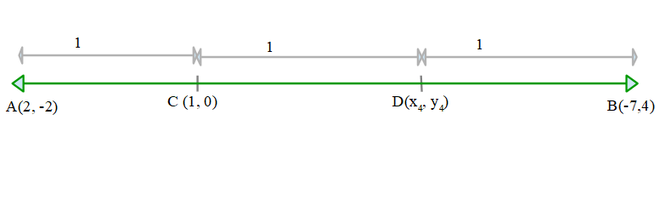D divides CB in ratio 1:1.

Now for section formula,

m =1, n = 1

x1 = -1, y1 = 0

x2 = -7, y2 = 4

Apply section formula:

x4 = (mx2 + nx1) /( m + n)

= ((1)(-7) + (1)(-1)) / (1 + 1)

= ( -7 -1 )/ 2

= -8/2

= -4

y4 = (my2 + ny1) / (m + n)

= ((1)(4) + (1)(0))/ (1 + 2)

= (4) / 2

= 2

Coordinate of D(x4 , y4) = ( -4, 2)

Therefore, (-1, 0) and (-4, 2) are the points of trisection of the line segment joining the points A(2, -2) and B(-7, 4).

### Sample Problems

Question 1: Find the coordinates of the points of trisection (i.e., points dividing into three equal parts) of the line segment joining the points A(0, 0) and B(0, 3).

Solution:

Let C and D be the point.

As AC = CD = DB then C will divide the line segment AB in 1:2 ratio.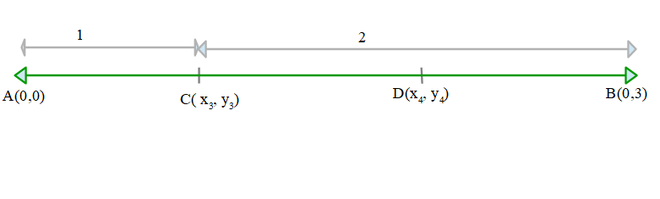Here,

m = 1, n= 2

x1 = 0, y1 = 0

x2 = 0, y2 = 3

Apply section formula:

x3 = (mx1 + nx1) / (m + n)

= ((1)(0) + (2)(0)) / (1 + 2)

= (0) / 3

= 0

y4 = (my1 + ny2) / (m + n)

= ((1)(3) + (2)(0)) / (1 + 2)

= (3) / 3

= 1

Coordinate of C(x3 , y3) = (0, 1)

Now using section formula or midpoint formula, calculate coordinate of D. D divides CB in ratio 1:1.

Now for section formula,

m =1, n = 1

x1 = 0, y1 = 1

x2 = 0, y2= 3

Apply section formula:

x4= (mx2 + nx1) / (m + n)

= ((1)(0) + (1)(0)) / (1+1)

= ( 0 )/ 2

= 0

y4= (my2 + ny1) / (m + n)

= ((1)(3) + (1)(1))/ (1 + 2)

= ( 4) / 2

= 2

Coordinate of D(x4 , y4) = ( 0, 2)

Question 2: Find the coordinates of the points that divide the line segment joining the points A(2, 4) and B(8,10) in 1:2 ratio.

Solution:

Let C be the point that divides the line segment joining the points A(2, 4) and B(8,10) in 1:2 ratio. Here,

m =1, n = 2

x1 = 2, y1 = 4

x2 = 8, y2 = 10

Apply section formula,

x= (mx2 + nx1) / (m + n)

= ((1)(8) + (2)(2)) / (1 + 2)

= (12 )/ 3

= 4

y = (my2 + ny1) / (m + n)

= ((1)(10) + (2)(4))/ (1 + 2)

= (18) / 3

= 6

Coordinate of point that divides line segment AB in 1:2 ratio is (4, 6).

My Personal Notes arrow_drop_up